# (CS 267, Apr 11 1995)

## Accelerating Graph Partitioning Using A Multilevel Approach

This material is based on "A fast multilevel implementation of recursive spectral bisection for partitioning unstructured problems" by Barnard and Simon, Proceedings of the 6th SIAM Conference on Parallel Processing for Scientific Computing, 1993.

The expensive part of spectral bisection is finding the eigenvector v2, which requires a possibly large number of matrix-vector multiplications with the Laplacian matrix L(G) of the graph G. To reduce the cost, we may use the following divide-and-conquer approach, which we will see later as well:

1. Approximate the initial graph G0=G by a simpler graph G1 with fewer nodes.
2. Partition G1, which should be cheaper since G1 is smaller.
3. Use the partition of G1 as an approximation for G0, which can be cheaply refined.
4. Apply the same algorithm recursively to partition G1, by finding a yet simpler graph G2, etc.

To make this work, we need to show how to extract G1 from G0, how to derive a partition of G0 from the partition of G1, and how to refine the approximate partitioning of G0 obtained from G1.

To get G1 from G0, we will eliminate nodes and edge from G0 while retaining the basic structure of G0. We do this by finding a maximal independent subset N1 of N0, where G0=(N0,E0). This means that

• N0 contains N1 and E0 contains E1,
• no nodes in N1 are directly connected by edges in E0 (independence), and
• N1 is as large as possible (maximality).
• There is a simple "greedy" algorithm for finding N1:
```   N1 = empty set
for i = 1 to |N0|
if node i is not adjacent to any node already in N1
end if
end for
```
This is shown below in the case where G0 is simply a chain of 9 nodes with nearest neighbor connections, in which case N1 consists simply of every other node of N1.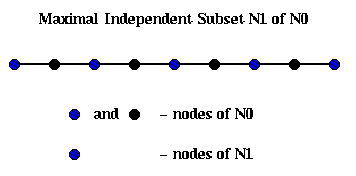To build G1=(N1,E1), we build E1 as follows: We will loop through the edges in E0, growing "domains" Di around each node i in N1. In other words, a domain consists of the subgraph of G0 connected to i by the edges examined so far. We will add an edge to E1 whenever an edge in E0 would connect two of these domains.

```   E1 = empty set
for all nodes i in N1
Di = ( { i }, empty set )         ... build initial domains
end for
unmark all edges in E0
repeat
choose an unmarked edge e=(i,j) from E0
if exactly one of i and j (say i) is in some Dk, then
add j and e to Dk
mark e
else if i and j are in different Dk's (say Dki and Dkj), then
add an edge (ki,kj) to E1
else if both i and j are in the same Dk, then
mark e
else
leave e unmarked
endif
until no unmarked edges
```

For example, suppose we start with G0 being a chain of nodes as above, with nodes and edges numbered from left to right. Then domain Di for node i will just contain its neighbor to the right, and the edges in E1 will simply connect adjacent nodes in N1, so G1 is just the chain of length n/2.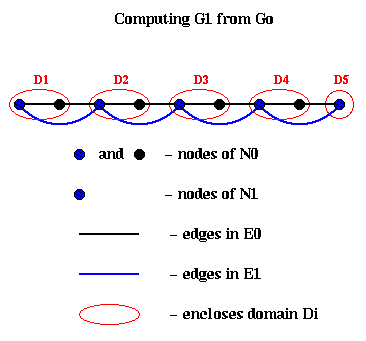To derive a partitioning of G1 from a partitioning of G0, we extract an approximate second eigenvector v2(G0) of G0 from v2(G1). This is done by interpolation:

```   for each node i in N0
if i is also a node in N1, then
v2(G0)(i) = v2(G1)(i), i.e. use the same eigenvector component
else
v2(G0)(i) = average of v2(G1)(j) for all neighbors j of i in N1.
end if
end for
```
This is shown below in the case of the chain of 9 nodes. The black line is the exact v2(G0) (normalized so the sum-of-squares is one). The dashed blue line is the exact v2(G1), also normalized, and which only connects every other node. The red +'s are the approximate v2(G0) computed by interpolation as above, which by construction lie on top of the blue line. The magenta x's are the red +'s normalized, to better see how well they approximate the black line.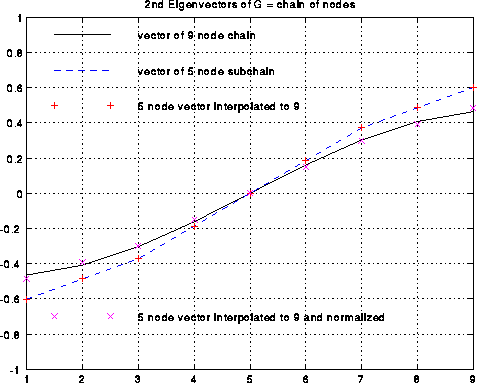Refining this approximate second eigenvector can be done in several ways. (The unrefined approximated second eigenvector is not always as good as in the case of the chain!). One possibility is that the Lanczos algorithm mentioned above benefits from having a starting vector which mostly points in the direction of the desired eigenvector. More aggressively, one can use a technique called Rayleight Quotient Iteration, which uses the fact that the iteration

```     choose a starting vector v(0)       ... v2(g1)
v(0) = v(0) / norm2(v(0))           ... norm2(x) = sqrt(sum_i x(i)^2)
i=0
repeat
i = i+1
rho(i) = v(i-1)' * L(G) * v(i-1)  ... rho(i) = Rayleigh Quotient
v(i) = inv( L(G) - rho(i)*I )* v(i-1)
v(i) = v(i) / norm2(v(i))
until convergence
```
converges asymptotically cubically to an eigenvalue-eigenvector pair (rho(i),v(i)). rho(i) costs one matrix-vector multiply. Computing v(i), i.e. solving the linear system (L(G) - rho(i)*I) * v(i) = v(i-1), is done using an iterative method (called SYMMLQ) which requires yet more matrix-vector multiplications. But since cubic convergence (cubing the error at every step) is so fast, very few steps are needed. This speedy convergence is illustrated below by the example of the chain, where the black line is the error in the approximate eigenvector v(i) and the dashed blue line is the error in the approximate eigenvalue rho(i), as functions of i. One can see the cubic convergence between iterations i=2 and i=3, where the eigenvector error goes from 1e-4 to (1e-4)^3 = 1e-12. Cubic convergence is only visible for this one step before hitting the accuracy limit of 10^(-16) due to roundoff.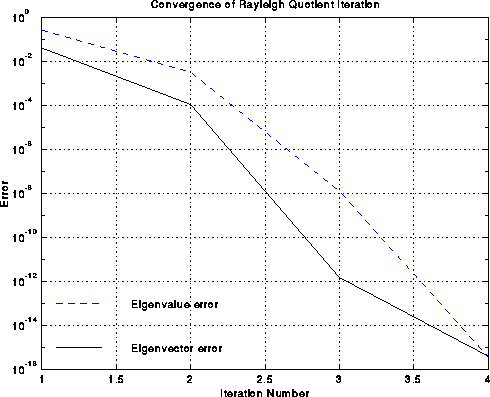Experiments report a 10x speedup over the basic spectral bisection algorithm.

An alternative divide-and-conquer approach, which does not use eigenvectors, is to simply use Kernighan-Lin to do the refinement. ("A multilevel algorithm for partitioning graphs", B. Hendrickson and R. Leland, Technical Report SAND93-1301, Sandia National Labs, Albuquerque NM, 1993).

## Performance Comparison of Different Partitioning Algorithms

This data is taken from "Geometric Mesh Partitioning" by J. Gilbert, G. Miller and S.-H. Teng (available from gilbert@parc.xerox.com).

Table 1 below describes the 7 graphs being partitioned. All are meshes in 2 or 3 dimensional space, rather than completely general graphs. The first four are 2-dimensional, and the last two are 3 dimensional. The fifth graph, PWT, is sometimes called "two-and-a-half dimensional", because it is a thin ("almost 2D") surface lying in 3D space. The last two columns give the number of vertices and edges in the graphs. The columns labeled "Grading" says how much larger the longest edges in the graph are than the shortest edges. For example, TRIANGLE is a regular tesselation of the place by identical equilateral triangles, so its grading is 1. AIRFOIL2 is similar to the NASA Airfoil we have seen so often, which has some large and some tiny triangles, the largest 1.3e5 times larger than the smallest.

```Mesh      Description              Mesh Type                  Grading  Vertices     Edges

TAPIR     Cartoon animal           2-D acute triangles        8.5e4       1024        2846
AIRFOIL2  Three-element airfoil    2-D triangles              1.3e5       4720       13722
TRIANGLE  Equilateral triangle     2-D triangles/same size    1.0e0       5050       14850
AIRFOIL3  Four-element airfoil     2-D triangles              3.0e4      15606       45878
PWT       Pressurized wind tunnel  Thin shell in 3-space      1.3e2      36519      144794
BODY      Automobile body          3-D volumes and surfaces   9.5e2      45087      163734
WAVE      Space around airplane    3-D volumes and surfaces   3.9e5     156317     1059331

Table 1:  Test problems.  "Grading" is the ratio of longest to shortest edge lengths.
```

Table 2 below describes the quality of the partitioning into two subgraphs obtained by four algorithms. Quality is measured by the number of edges crossing the partition boundary, where fewer is better. The four algorithms are

1. Spectral -- use the second eigenvector as described above
2. Inertial Partitioning -- coordinate bisection as described in Lecture 18
3. Default Random Circle -- as described in Lecture 18. Recall that this is a randomized algorithm, that involves picking a random circle. The more circles chosen, the better the partitioning (i.e. the fewer edges cut). In this default implementation, a small, fixed number of circles are chosen.
4. Best Random Circle -- In this case circles are repeatedly chosen until no more progress is made. It is more expensive than the Default Random Circle algorithm just described, but gives a better partitioning.
From the table, one sees that the methods are largely comparable, with spectral somewhat better on the largest graphs. (Spectral is also much more expensive to run, although we present no data on this here.) Also, we see that our intuition, that a 2D mesh-like graph with n nodes should have a partition with just sqrt(n) edge crossings, is approximately true. Also, our intuition that a 3D mesh should have n^(2/3) edge crossings is also approximately true.
```Mesh          Spectral           Inertial        Default           Best
Partitioning   Random Circle   Random Circle

TAPIR            59                55                37              32
AIRFOIL2        117               172               100              93
TRIANGLE        154               142               144             142
AIRFOIL3        174               230               152             148
PWT             362               562               529             499
BODY            456               953               834             768
WAVE          13706              9821             10377            9773

Table 2:  Number of edge crossings for two-way partitions
```

Finally, we use the partitioning algorithms 7 times recursively in order to partition the graphs into 2^7 = 128 separate partitions, which one would do on a 128-processor machine. Again, the quality of the partitions is largely comparable.

```Mesh          Spectral           Inertial        Default
Partitioning   Random Circle

TAPIR           1278              1387              1239
AIRFOIL2        2826              3271              2709
TRIANGLE        2989              2907              2912
AIRFOIL3        4893              6131              4822
PWT            13495             14220             13769
BODY           12077             22497             19905
WAVE          143015            162833            145155

Table 3:  Number of edge crossings for 128-way partitions.
```

## Applying Spectral Bisection to DNA Sequencing

The following material is based on "A spectral algorithm for the seriation problem", by J. Atkins, E. Boman and B. Hendrickson (bah@cs.sandia.gov), submitted to FOCS 95.

Here is a very simple version of the DNA sequencing problem. A molecule of DNA is a very long string consisting of a particular sequence of amino acids chosen from a set of four, which may be called A, D, T and G. For example, one might have the sequence ADDTGADTDGAGADTDG, but many millions long. The sequencing problem is to determine this sequence for a given molecule of DNA. Current biochemical technology permits the following algorithm to be used. One can break up this long DNA string into a great many shorter fragments, which can be separated according to their amino acid sequences. The goal is to represent the original DNA as a sequence of these possibly overlapping fragments in some order. Given the sequences making up each fragment, one then knows the sequence making up the original DNA. To extract this representation in terms of fragments, the following experiments are performed. Each fragment F is allowed to bond to the DNA at a point P where there amino acid sequences match. This point is called a probe, and is typically a set of amino acids at one end of the fragment. If the probe is long enough, it will match at a unique point along the DNA. One records this information in a matrix B, which has one row for each fragment and one column for each probe, by putting a 1 at entry (F,P). One can perform the same kind of experiment to see which other fragments bond to fragment F at the same probe P, implying that they overlap. Each such bond between fragment F' and fragment F at probe P is recorded by storing a 1 at location (F',P) of the matrix B. In this way, looping through all the fragments, the matrix B is eventually filled in with ones (and zeros elsewhere), where B(F,P)=1 means that fragment F matches the DNA at probe P. This is shown below, where we have labeled the fragments in sorted order from left to right, and the probes in sorted order from left to right.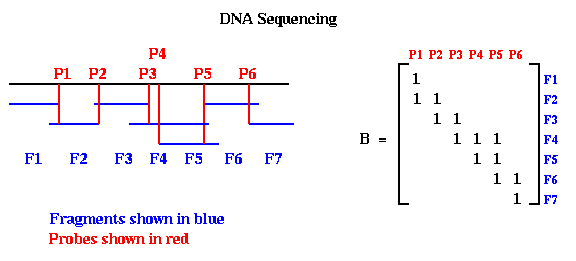Notice that when the probes and fragments are sorted, B is a band matrix, which means all its nonzero entries are near the diagonal. In practice, one constructs Bp with probes and fragments in some random order, as shown below. The DNA Sequencing problem is to find the ordering of the rows and ordering of the columns of Bp which expose the underlying band matrix B, because this will say what in what order the fragments appear along the DNA.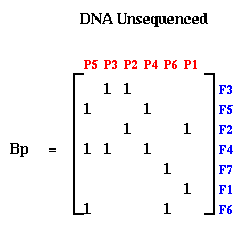If there were no errors in Bp, this could be done by procedure like breadth first search. But in practice, the laboratory procedure for determining entries of Bp is quite error prone, so we need a method for making Bp "close to" a band matrix in some sense. This is where spectral bisection comes in.

Let G=(N,E) be an undirected graph, and L(G) its Laplacian. Let N = N- U N+ be an arbitrary partition of the nodes, and let x be a column vector, where x(i) = +1 if i is in N+, and x(i) = -1 if i is in N-. In Lemma 1 of the last lecture, we showed that the number of edges connecting N+ and N- was equal to

```   .25 * sum_{edges e=(i,j)} (x(i) - x(j))^2
```
Therefore, the partition N = N- U N+ which minimizes the number of connecting edges is given by the solution x of the minimization problem
```     min_{x(i) = +1 or -1, sum_i x(i) = 0} .25 * sum_{edges e=(i,j)} (x(i) - x(j))^2
```
The spectral partitioning algorithm involved solving the following approximation:
```     min_{sum_i v(i)^2 = |N|, sum_i v(i) = 0} .25 * sum_{edges e=(i,j)} (v(i) - v(j))^2
```
and then choosing x(i) = sign(v(i)).

One can think of this algorithm as embedding the graph G into the real axis, putting node i at location v(i). If e=(i,j) is an edge, then we draw a line segment from v(i) to v(j), which has length |v(i) - v(j)|. The spectral bisection algorithm chooses this embedding into the real axis so as to minimize the sum of squares of the lengths of these line segments, subject to the constraints sum_i v(i)^2 = |N|, and sum_i v(i)=0.

Suppose we start with a symmetric matrix H, form it graph G(H), and apply the above algorithm, yielding a second eigenvector v of L(G(H)). Let P be a permutation matrix, i.e. the identity matrix with its columns permuted, such that the entries of P*v, which are the the entries of v permuted the same way, are in sorted order. Now form N = P*H*P'. N is the matrix H with its rows and columns reordered in the same way that sorts v. The fact that the sum of all (v(i)-v(j))^2 is minimized, means that there are few edges connecting distant v(i) and v(j). In other words, if v(i) and v(j) are widely separated in the sorted list of entries of v, they are unlikely to have an edge connecting them. In the matrix N, this means that there are few nonzero entries far from the diagonal, because these would correspond to an edge from a v(i) to a v(j) widely separated in the sorted list. In other words, N is close to a band matrix.

For example, consider the matrix M = L(G), where G is a chain of n nodes. M is a tridiagonal matrix as shown in the figure below. Now perform a random permutation of the rows and columns of M to get H. H has nonzeros uniformly distributed off the diagonal. Apply spectral partitioning to H as described above to get N. As shown below, N is tridiagonal again. (The label nz under each graph is the number of nonzeros entries in the matrix.)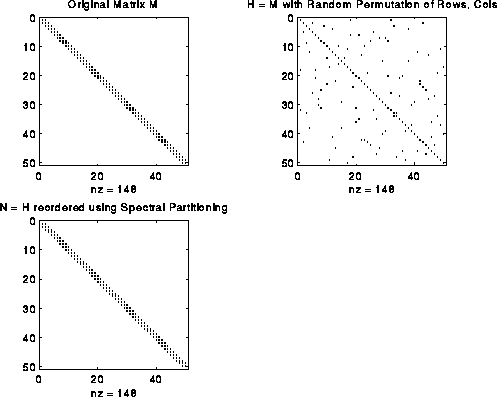This bandwidth narrowing property is what we need to reorder the rows and columns of Bp to make it a band matrix. But reordering Bp requires two permutations, one for the rows and one for the columns, while spectral bisection computes just one permutation. We get around this as follows. We can write Bp = Pf*B*Pp', where Pf and Pp are two unknown permutation matrices we wish to compute; Pf shuffles the rows of B, and Pp shuffles the columns. Now consider the symmetric matrix

```     Tp = Bp'*Bp = (Pf*B*Pp')'*(Pf*B*Pp') = Pp*(B'*B)*Pp'
```
Note that Tp only depends on Pp and B, but not on Pf. If B is a band matrix, one can confirm that B'*B is too, although with a larger bandwidth. Thus, Pp can be determined just by using spectral bisection to find the single permutation of rows and columns of Tp that makes Pp'*Tp*Pp (nearly) a band matrix. Similarly one can apply spectral bisection to
```     Tf = Bp*Bp' = (Pf*B*Pp')*(Pf*B*Pp')' = Pf*(B*B')*Pf'
```
to independently determine Pf. An example is shown below, where we start with a perfect band matrix and add a few other random entries to get B, and randomly permute its rows and columns to get Bp. The bottom row of three matrices shows Tp, Tf and Bp after permuting them to make them close to band matrices. One can see that the construction is far from "perfect", and in fact degrades more if the random entries added to B are farther from the diagonal. DNA sequencing remains a hard problem.## Software for Graph Partitioning

Software is available for all the partitioning methods described here from various sources.

Chaco was written by Bruce Hendrickson and Robert Leland at Sandia National Lab. It is a serial library containing implementations of many of the methods we have discussed. A manual for v. 1.0 is in the class reader ("The Chaco User's Guide, Version 1.0", Technical Report SAND93-2339, Sandia National Labs, Albuquerque NM 1993). It is available electronically from the authors (rwlelan@cs.sandia.gov or bahendr@cs.sandia.gov). It is freely available for research purposes.

JOSTLE was written by C. Walshaw, M. Cross, and M. Everett at the University of Greenwich in the UK. It is available free to researchers, but a license agreement is involved. Further information is available here, and the license agreement is here.

The software for the geometric partitioning algorithm by Gilbert, Miller, Teng, Vavasis and Thurston is written in matlab, and can be obtained by anonymous ftp from machine ftp.parc.xerox.com as file /pub/gilbert/mashpart.uu.

The above software consists of libraries to which one passes a graph, and is returned a partitioning. There have also been attempts to embed graph partitioning in a higher level language, so as shield the user from having to construct the graph, partition it, (re)distribute the data across the machine, and set up the communication. The goal of this work is to be able to take an existing serial code which traverses a sparse data structure, and modify the language and compiler to permit the user to say

1. Inspect the following section of code (a loop nest, say), and determine the underlying graph G describing how data items depend on other data items, partition G, and redistribute the data accordingly.
2. Execute the code with the redistributed data.

The system we will describe is called PARTI, which stands for Parallel Automated Runtime Toolbox at ICASE, where ICASE is a NASA computing research laboratory. The primary author is Joel Saltz, currently at the University of Maryland at College Park. This materal is taken from "Distributed Memory Compiler Methods for Irregular Problems -- Data Copy Reuse and Runtime Partitioning," by R. Das, R. Ponnusamy, J. Saltz and D. Mavripilis, ICASE Report 91-73, NASA Langley Research Center, Hampton VA, 1991. This report is in the class reader.

PARTI is an extension of HPF (High Performance Fortran), and uses the features of HPF for describing array layouts across processors. We begin by reviewing data layouts.

At the end of Lecture 5, we discussed data layout in CM Fortran, where for example the declaration (KEYWORDS are capitalized)

```      REAL a(64,8), b(64,8), c(64,8)
CMF\$  LAYOUT a( :NEWS, :SERIAL ), b( :NEWS, :SERIAL ), c( :SERIAL, :NEWS )
```
indicated that A(i,j) was to be stored in the j-th memory location of processor i, the same for B(i,j), and that C(i,j) was instead to be stored in the i-th memory location of processor j. This would mean that the assignment A=B could occur in parallel without communication, but that A=C would require a great deal of communication.

These simple layout directive are not enough for all purposes. In the beginning of Lecture 13, we discussed the more complicated data layouts required to do Gaussian elimination (or other dense linear algebra problems) efficiently on a distributed memory machine, and said the the first four of the following layouts were declarable within the HP Fortran language: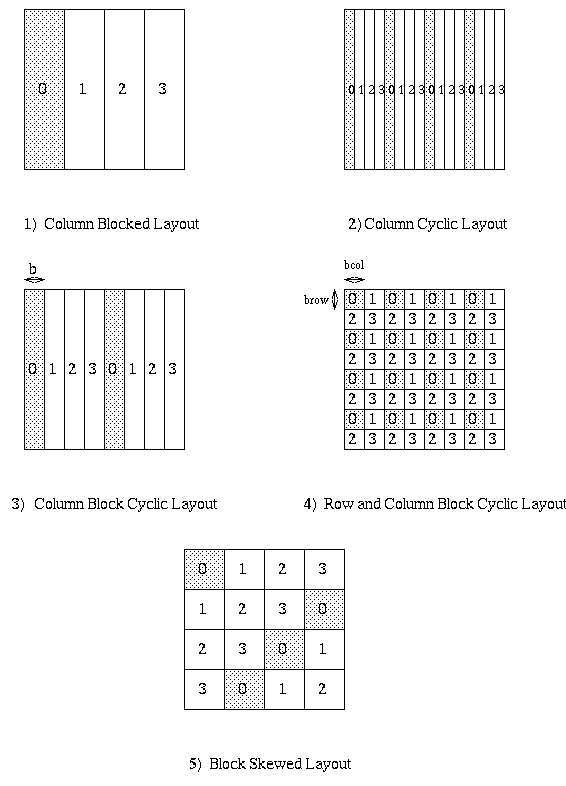Here, very briefly, is how HPF permits users to declare these kinds of layouts. Rather than saying how each matrix entry maps to a processor location, two levels of indirection are used. The first level declaration declares how many of the available processors are to be used in the layout. A simple example is the following, which declares mygrid to be a linear array of 4 processors.

```     PROCESSOR mygrid(4)
```
The second level declares a template, or "virtual array", and says how to lay it out on mygrid. For example
```     TEMPLATE template_blocked(100),template_cyclic(100)
DISTRIBUTE template_blocked(BLOCK) ONTO mygrid
DISTRIBUTE template_cyclic(CYLIC) ONTO mygrid
```
declares that template_blocked(0:24) is mapped to processor 0, template_blocked(25:49) is mapped to processor 1, and so on, in general with template_blocked(i) mapping to processor floor(i/25). Also, template_cyclic(i) is mapped to processor i mod 4. Block cyclic layouts are also available. Multi-dimensional arrays can have each subscript mapped independently, as preferred for Gaussian Elimination.

A template has no memory allocated for it; it just describes a layout. The final level of declaration actually allocates memory for arrays. For example

```     REAL  a(100), b(100), c(100)
ALIGN a(i) WITH template_block(i)
ALIGN b(i) WITH template_block(i)
ALIGN c(i) WITH template_cyclic(i)
```
declares 3 arrays of 100 entries each. a(i) and b(i) are declared to be stored at the same place as the template entry template_block(i), in this case floor(i/25). However template_block is DISTRIBUTEd, a(i) and b(i) will always be on the same processor. c(i) is declared to be stored at the same place as template_cyclic(i), that is i mod 4.

The reason for these levels of indirection is that one can independently control the amount of parallelism (via PROCESSOR), the layout (via DISTRIBUTE) and which variables are local with which other (via ALIGN).

The same mechanism can be used for more irregular layouts, but we need one more level of indirection to specify the irregularity. For example

```     TEMPLATE irregular(100)
INTEGER map(100)
DATA map/3,2,2,1,1,1,3,0,1,2,... /           ... 100 values from 0 to 3
DISTRIBUTE irregular(map) ONTO mygrid
REAL d(100)
ALIGN d(i) WITH irregular(i)
```
These declarations specify that irregular(i) is mapped to processor map(i), i.e. irregular(1) is mapped to processor map(1)=3, irregular(2) is mapped to processor map(2) = 2, and so on. The ALIGN statement in turn says d(1) is stored on processor 3, d(2) is stored on processor 2, and so on.

In this example, map is specified at compile-time, and the decision about where to store d(i) is specified at compile-time. This is very limiting, since we probably won't know the actual data structure we need to partition until run-time. The extensions in PARTI to this approach are to allow map to be computed at run time (by examining some user specified loops and doing graph partitioning), and DISTRIBUTE to be executed at run-time as well, in effect recompiling the code at run-time. This is likely to be quite expensive, and so is done only when the user wants to.

Here is an example, taken from a computational fluid dynamic (CFD) application. The data structure is a two-dimension triangular mesh, made up of nodes (numbered in blue), edges (numbered in black) and faces (numbered in red). The mesh data is stored in two arrays. The edge_list array stores a pair of nodes for each each edge: the nodes numbers for the i-th edge are stored at edge_list(i) and edge_list(i + n_edge), where n_edge is the number of edges. The face_list array stores a triple of nodes for each face: the nodes for the i-th face are stored at face_list(i), face_list(i + n_face), and face_list(i + 2*n_face), as shown below. For example, face 1 has corners at nodes 1, 2 and 3. face 2 at nodes 2, 3 and 4, and so on.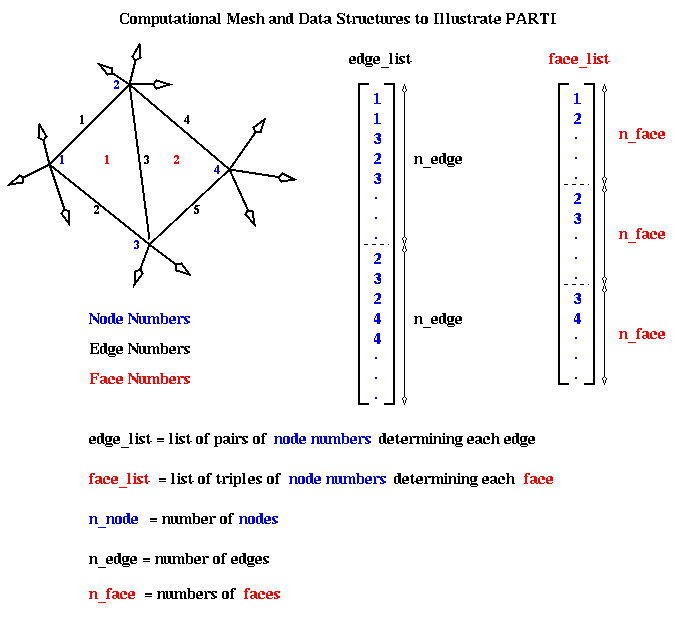The original sequential program has two data arrays, x and y, which store data associated with each node. In other words x(i) and y(i) are data about the fluid flow at node i. The algorithm has two loops. Loop L1 below loops over all edges, and for each edge updates the data at both nodes determining that edge. Loop L2 loops over all faces, and for each face updates the data at the three nodes determining the face. The functions foo1, foo2, etc, are simple scalar functions of their scalar arguments, whose details do not concern us.

```    REAL x(n_node), y(n_node)
...
C   Loop over all edges
L1: DO i = 1, n_edge
n1 = edge_list(i)
n2 = edge_list(i + n_edge)
y(n1) = foo1(y(n1),y(n2),x(n1),x(n2))
y(n2) = foo2(y(n1),y(n2),x(n1),x(n2))
END DO

C   Loop over all faces
L2: DO i = 1, n_face
n1 = face_list(i)
n2 = face_list(i + n_face)
n3 = face_list(i + 2*n_face)
y(n1) = foo4(y(n1),...,x(n3))
y(n2) = foo5(y(n1),...,x(n3))
y(n3) = foo6(y(n1),...,x(n3))
END DO
```

Here is the parallel version of this program using PARTI:

```    REAL x(n_node), y(n_node)
TEMPLATE coupling(n_node)
DISTRIBUTE coupling(BLOCK) ONTO mygrid
ALIGN x(i), y(i) WITH coupling(i)
...
C   Decide whether to redistribute data
IF (time_to_remap) THEN
DISTRIBUTE coupling(IMPLICIT USING L1)
END IF
C   Loop over all edges

IMPLICITMAP(x,y) L1
L1: DO i = 1, n_edge
n1 = edge_list(i)
n2 = edge_list(i + n_edge)
y(n1) = foo1(y(n1),y(n2),x(n1),x(n2))
y(n2) = foo2(y(n1),y(n2),x(n1),x(n2))
END DO

C   Loop over all faces
L2: DO i = 1, n_face
n1 = face_list(i)
n2 = face_list(i + n_face)
n3 = face_list(i + 2*n_face)
y(n1) = foo4(y(n1),...,x(n3))
y(n2) = foo5(y(n1),...,x(n3))
y(n3) = foo6(y(n1),...,x(n3))
END DO
```

Initially, the TEMPLATE coupling is laid out in a blocked fashion onto the processor grid mygrid. Arrays x and y are aligned with coupling. Later, perhaps after the arrays edge_list and face_list have been set up, the user sets time_to_remap to true, and executes the DISTRIBUTE statement following the IF statement. At this point, the system begins the "Inspection phase": It will examine loop L1, which is identified later with the IMPLICITMAP statement. The arguments x and y of IMPLICITMAP tell the system to compute the graph G of data dependencies among the references to x and y in the subsequent loop L1. This is done by executing loop L1 "symbolically", i.e. running through thg loop from i=1 to n_edge, computing the subscripts n1 = edge_list(i) and n2 = edge_list(i + n_edge), seeing that y(n1) depends on y(n1), y(n2), x(n1), and x(n2), and adding nodes n1 and n2, and edge (n1,n2), to graph G, which is initially empty. Functions foo1 and foo2 are not evaluated, and y(n1) and y(n2) are not changed. After computing G, a graph partitioning routine is called to break G into as many pieces as there are processors in mygrid. The partitioning information is stored in the TEMPLATE coupling, with coupling(i) = j if the partition algorithm puts node i onto processor j. All arrays ALIGNed with coupling (namely x and y) are redistributed according to the newly upated coupling. Finally, all parts of the program that reference arrays x or y are "recompiled" to insert the necessary communications to continue to access the data they need.

After completing the "IF (time_to_remap)" block, one reaches loop L1. At this point, the "Execute phase", the newly recompiled code is executed and array y updated. Loop L2 is executed similarly.